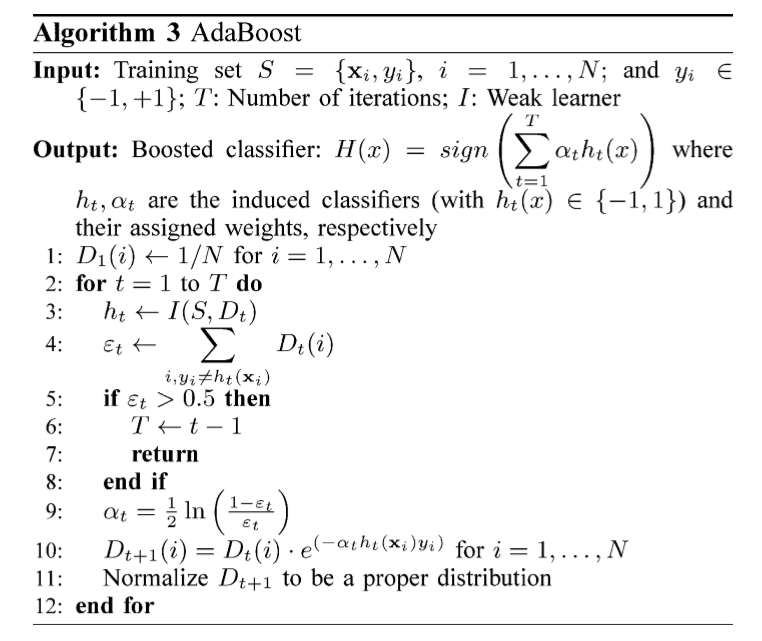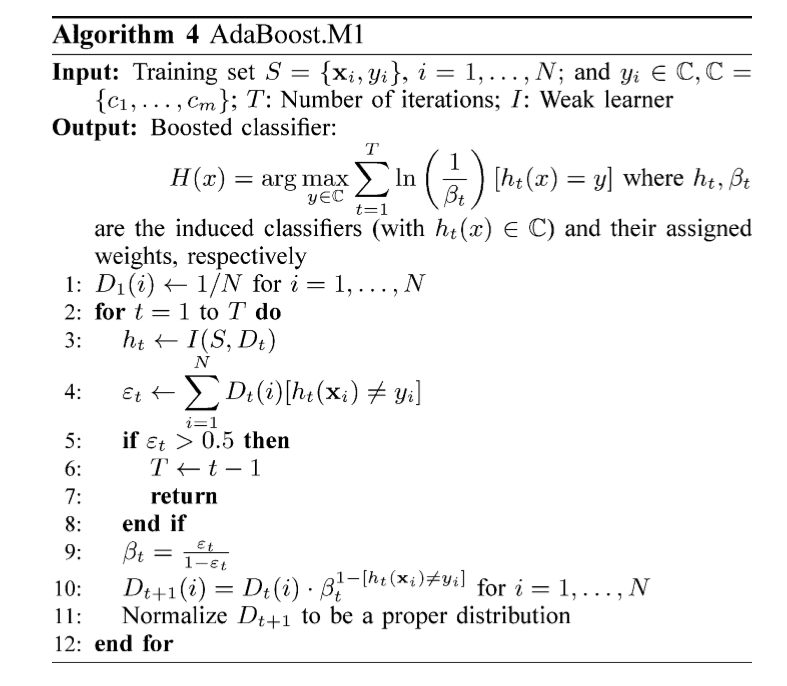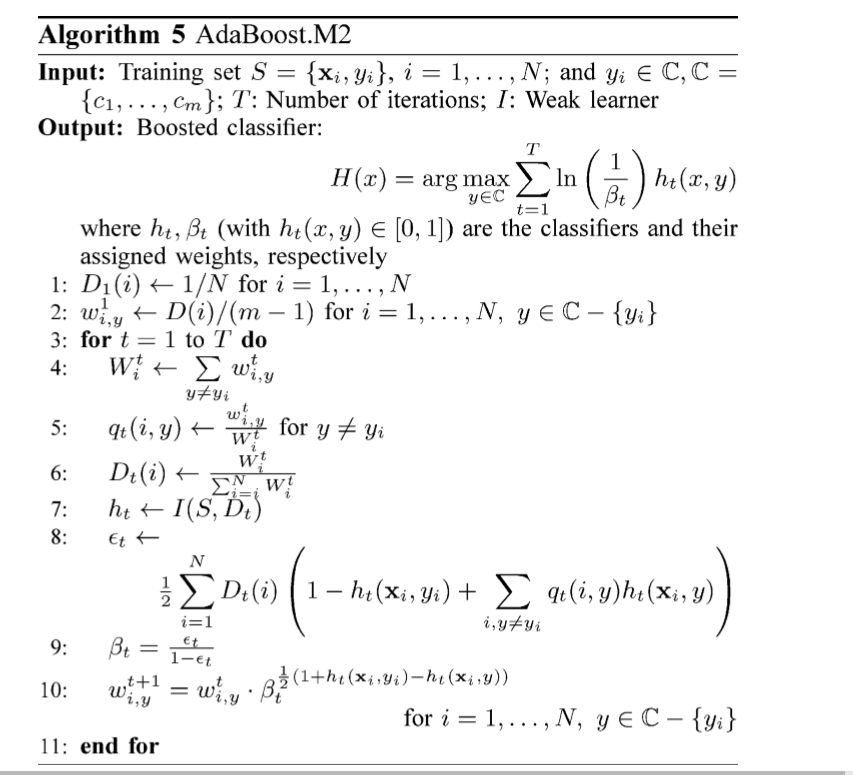# 三种算法的差别H(x)=sign(t=1Tαtht(x))

Dt+1(i)={Dt(i)eαtDt(i)eαtif ht(xi)=yi,if not.(1)# 一

H(x)$H(x)$的形式发生了一些变化，没有使用符号函数sign()$sign()$,但作用并没有发生实质的变化，任然可以看成是及基分类器对输出结果进行投票，而且基分类器的权重实质上也没有变化，依然是ln(1ϵtϵt)$ln(\frac{1-\epsilon_t}{\epsilon_t} )$

## 二wti,y$w_{i,y}^t$表示第t次迭代中样本i的某个错误标签y的权重

Wti$W_i^t$表示在第t次迭代中样本i的的错误标签的权重和

qt(i,y)$q_t(i,y)$表示wti,y$w_{i,y}^t$Wti$W_i^t$中占的比重

Dt(i)$D_t(i)$表示第i个样本在所有样本中占的权重©️2019 CSDN 皮肤主题: 大白 设计师: CSDN官方博客